Courses

# Divide And Conquer (Advance Level) - 1

## 15 Questions MCQ Test Question Bank for GATE Computer Science Engineering | Divide And Conquer (Advance Level) - 1

Description
This mock test of Divide And Conquer (Advance Level) - 1 for Computer Science Engineering (CSE) helps you for every Computer Science Engineering (CSE) entrance exam. This contains 15 Multiple Choice Questions for Computer Science Engineering (CSE) Divide And Conquer (Advance Level) - 1 (mcq) to study with solutions a complete question bank. The solved questions answers in this Divide And Conquer (Advance Level) - 1 quiz give you a good mix of easy questions and tough questions. Computer Science Engineering (CSE) students definitely take this Divide And Conquer (Advance Level) - 1 exercise for a better result in the exam. You can find other Divide And Conquer (Advance Level) - 1 extra questions, long questions & short questions for Computer Science Engineering (CSE) on EduRev as well by searching above.
QUESTION: 1

### Choose the false statements:

Solution:

Internal sorting is such a process that takes place entirely within the main memory of a computer. This is only possible whenever data items, to be sorted, is small enough to be accommodated in main memory. Hence, internal sorting do not need auxiliary storage.
External sorting is just opposite to interval sorting and used when input data, to be sorted, is very large. Hence, external sorting need auxiliary storage.

QUESTION: 2

### A sorting technique that guarantees, that records with the same primary key occurs, in the same order in the sorted list as in the original unsorted list is said to be

Solution:

A sorting algorithm is said to be stable sorting algorithm if two objects /records with equal primary key appears in the same order in the sorted list as in the original unsorted list.

QUESTION: 3

### Randomized quicksort is an extension of quicksort where the pivot is chosen randomly. What is the worst case complexity of sorting n numbers using randomized quicksort?

Solution:

Randomized quick sort worst case time complexity = O (n2).

QUESTION: 4

Consider a list of recursive algorithms and a list of recurrence relations as shown below. Each recurrence relation corresponds to exactly one algorithm and is used to derive the time complexity of the algorithm.

List-I (Recursive Algorithm)
P. Binary search
Q. Merge sort
R. Quicksort
S. Tower of Hanoi
List-li (Recurrence Relation)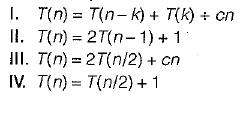Which of the following is the correct match between the algorithms and their recurrence relations?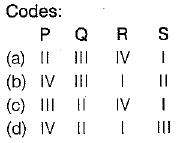Solution:

Binary search : T(n) = T(n/2) + 1
Merge sort : T(n) = 2T(n/2) + cn
Quick sort : T(n) = T(n - k) + T(k) + cn

Tower of Hanoi : T(n) = 2T(n - 1) + 1

QUESTION: 5

Which of the following sorting algorithms has the lowest worst-case complexity?

Solution: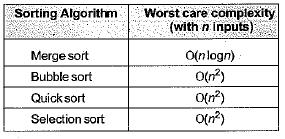QUESTION: 6

In the following C function, let n ≥ m.
int gcd(n, m)
{
if (n% m = - 0} return m;
n = n% m;
return gcd (m, n);
}
How many recursive calls are made by this function?

Solution:

Let, T(m, n) be the total number of steps.
So, T(m, 0) = 0, T{m, n) = T{n, m m o d n ) on average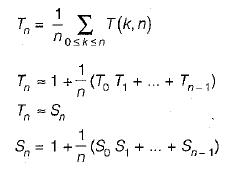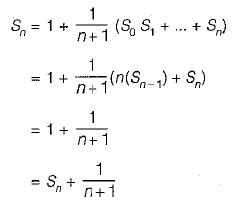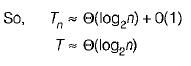QUESTION: 7

In quick sort, for sorting n elements, the (n/4)th smallest element is selected as pivot using an O(n) time algorithm. What is the worst case time complexity of the quick sort?

Solution:

The relation T(n) = T(n/4) + T(3n/4) + n The pivot element is selected in such a way that it will divide the array into 1/4th and 3/4th always solving this relation give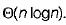QUESTION: 8

The worst case running time to search for an element in a balanced binary search tree with n 2n elements is

Solution:

We know that in balanced BST there are log2n levels in both worst as well as best case where ‘n’ is number of elements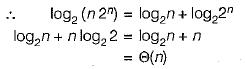QUESTION: 9

A list of n strings, each of length n, is sorted into lexicographic order using the merge sort algorithm. The worst case running time of this computation is

Solution:

The recurrence tree for merge sort will have height n and O (n2) work will be done at each level of the recurrence tree (Each level involves ncomparisons and a comparison takes O(n) time in worst case). So time complexity of this merge sort will be O(n2 logn).

QUESTION: 10

Which one of the following is the tightest upper bound that represents the time complexity of inserting an object into a binary search tree of n nodes?​

Solution:

In the worst case length of binary search tree can be O(n).
So insertion of an object will take O(n) time in worst case.

QUESTION: 11

Which one of the following is the recurrence equation for the worst case time complexity of the Quicksort algorithm for sorting n(≥ 2) numbers? In the recurrence equations given in the options below, c is a constant.

Solution:

In worst case the pivot element position may be either first or last. In that case the elements are always divided in 1 : (n - 1) proportion. The recurrence relation for such a proportional division would be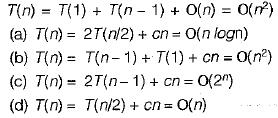QUESTION: 12

What are the worst-case complexities of insertion and deletion of a key in a binary search tree?

Solution:

In worst case the BST may be Skewed BST. This case occurs when we insert the elements in increasing order or decreasing order. Example: Consider we insert elements, in the order 1, 12, 39, 43, 50......
The BST would be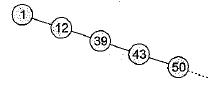To find ‘n’ is the worst case we may have to traverse to bottom of tree which takes O(n) time.
Hence for both insertion and deletion worst case goes to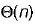QUESTION: 13

The average number of comparisons performed by the merge sort algorithm, in merging two sorted lists of length 2 is

Solution:

Size sorted list has size 2, So, number of comparison is between min (2,2) to (2 + 2 - 1) = 3 So, 2, 3, 3 are number of comparisons.
So average number of comparison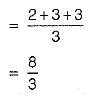QUESTION: 14

Pick the correct statements:

Solution:

Sequential to be organization suitable for batch processing and not suitable for interactive processing.

QUESTION: 15

Which of the following statements is true?
1. As the number of entries in the hash table increases, the number of collisions increases.
2. Recursive program are efficient.
3. The worst time complexity of quick sort is O(n2).
4. Binary search implemented using a linked list is efficient

Solution:

Recursive programs take more time than the equivalent non-recursive version and so not efficient. This is because of the function call overhead.
In binary search, since every time the current list is probed at the middle, random access is preferred. Since linked list does not support random access, binary search implemented this way is inefficient.